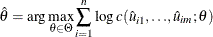The COPULA Procedure

Canonical Maximum Likelihood Estimation (CMLE)

In the canonical maximum likelihood estimation (CMLE) method, it is assumed that the sample data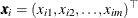,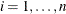have been transformed into uniform variates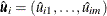,. One commonly used transformation is the nonparametric estimation of the CDF of the marginal distributions, which is closely related to empirical CDF,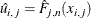where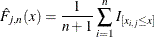The transformed data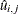are used as if they had uniform marginal distributions; hence, they are called pseudo-samples. The function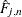is different from the standard empirical CDF in the scalar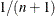, which is to ensure that the transformed data cannot be on the boundary of the unit interval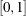. It is clear that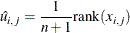where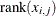is the rank amongin increasing order.

Let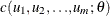be the density function of a copula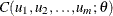, and letbe the parameter vector to be estimated. The parameteris estimated by maximum likelihood: